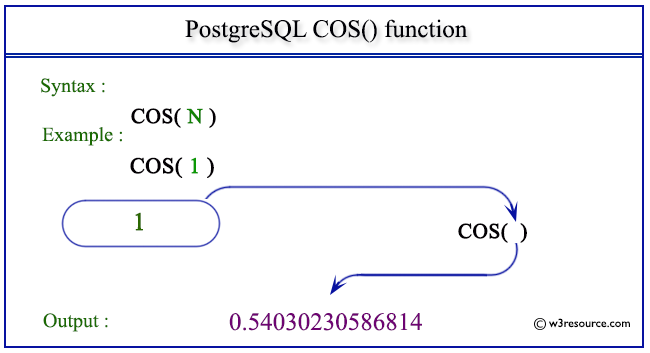# PostgreSQL COS() function

## COS() function

The PostgreSQL cos() function is used to return the cosine of a given argument.

Syntax:

```cos(a)
```

PostgreSQL Version: 9.3

Pictorial presentation of PostgreSQL COS() functionExample PostgreSQL COS() function

Code:

``````SELECT COS(0) AS "Cos (0)";
```
```

Sample Output:

``` Cos (0)
---------
1
(1 row)
```

Example 2: PostgreSQL COS() function

Code:

``````SELECT COS(1) AS "Cos (1)";
```
```

Sample Output:

```     Cos (1)
------------------
0.54030230586814
(1 row)
```

Example 3: PostgreSQL COS() function

Code:

``````SELECT COS(-1) AS "Cos (-1)";
```
```

Sample Output:

```     Cos (-1)
------------------
0.54030230586814
(1 row)
```

Previous: CEILING function
Next: COT function

﻿